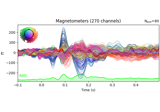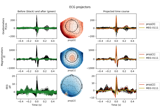# mne.preprocessing.compute_proj_eog#

mne.preprocessing.compute_proj_eog(raw, raw_event=None, tmin=-0.2, tmax=0.2, n_grad=2, n_mag=2, n_eeg=2, l_freq=1.0, h_freq=35.0, average=True, filter_length='10s', n_jobs=None, reject={'eeg': 0.0005, 'eog': inf, 'grad': 2e-10, 'mag': 3e-12}, flat=None, bads=[], avg_ref=False, no_proj=False, event_id=998, eog_l_freq=1, eog_h_freq=10, tstart=0.0, filter_method='fir', iir_params=None, ch_name=None, copy=True, return_drop_log=False, meg='separate', verbose=None)[source]#

Compute SSP (signal-space projection) vectors for EOG artifacts.

This function will:

1. Filter the EOG data channel.

2. Find the peaks of eyeblinks in the EOG data using `mne.preprocessing.find_eog_events()`.

3. Filter the raw data.

4. Create `Epochs` around the eyeblinks.

5. Optionally average the `Epochs` to produce an `Evoked` if `average=True` was passed (default).

6. Calculate SSP projection vectors on that data to capture the artifacts.

Note

Parameters:
raw`mne.io.Raw`

Raw input file.

raw_event

Raw file to use for event detection (if None, raw is used).

tmin`float`

Time before event in seconds.

tmax`float`

Time after event in seconds.

n_grad`int`

Number of SSP vectors for gradiometers.

n_mag`int`

Number of SSP vectors for magnetometers.

n_eeg`int`

Number of SSP vectors for EEG.

l_freq

Filter low cut-off frequency for the data channels in Hz.

h_freq

Filter high cut-off frequency for the data channels in Hz.

averagebool

Compute SSP after averaging. Default is True.

filter_length

Number of taps to use for filtering.

n_jobs

The number of jobs to run in parallel. If `-1`, it is set to the number of CPU cores. Requires the `joblib` package. `None` (default) is a marker for ‘unset’ that will be interpreted as `n_jobs=1` (sequential execution) unless the call is performed under a `joblib.parallel_config` context manager that sets another value for `n_jobs`.

reject

Epoch rejection configuration (see Epochs).

flat

Epoch flat configuration (see Epochs).

bads`list`

avg_refbool

no_projbool

Exclude the SSP projectors currently in the fiff file.

event_id`int`

ID to use for events.

eog_l_freq`float`

Low pass frequency applied to the E0G channel for event detection.

eog_h_freq`float`

High pass frequency applied to the EOG channel for event detection.

tstart`float`

Start artifact detection after tstart seconds.

filter_method`str`

Method for filtering (‘iir’ or ‘fir’).

iir_params

Dictionary of parameters to use for IIR filtering. See mne.filter.construct_iir_filter for details. If iir_params is None and method=”iir”, 4th order Butterworth will be used.

ch_name

If not None, specify EOG channel name.

copybool

If False, filtering raw data is done in place. Defaults to True.

return_drop_logbool

If True, return the drop log.

New in v0.15.

meg`str`

Can be ‘separate’ (default) or ‘combined’ to compute projectors for magnetometers and gradiometers separately or jointly. If ‘combined’, `n_mag == n_grad` is required and the number of projectors computed for MEG will be `n_mag`.

New in v0.18.

verbose

Control verbosity of the logging output. If `None`, use the default verbosity level. See the logging documentation and `mne.verbose()` for details. Should only be passed as a keyword argument.

Returns:
projs

List of computed projection vectors.

eog_events: `ndarray`

Detected EOG events.

drop_log`list`

The drop log, if requested.

Notes

Filtering is applied to the EOG channel while finding events using `eog_l_freq` and `eog_h_freq`, and then to the `raw` instance using `l_freq` and `h_freq` before creation of the epochs used to create the projectors.

## Examples using `mne.preprocessing.compute_proj_eog`#Working with CTF data: the Brainstorm auditory dataset

Working with CTF data: the Brainstorm auditory datasetRepairing artifacts with SSP

Repairing artifacts with SSP